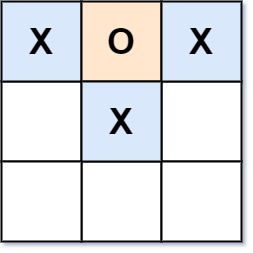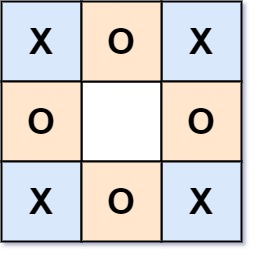## Algorithm

Problem Name: 794. Valid Tic-Tac-Toe State

Given a Tic-Tac-Toe board as a string array `board`, return `true` if and only if it is possible to reach this board position during the course of a valid tic-tac-toe game.

The board is a `3 x 3` array that consists of characters `' '`, `'X'`, and `'O'`. The `' '` character represents an empty square.

Here are the rules of Tic-Tac-Toe:

• Players take turns placing characters into empty squares `' '`.
• The first player always places `'X'` characters, while the second player always places `'O'` characters.
• `'X'` and `'O'` characters are always placed into empty squares, never filled ones.
• The game ends when there are three of the same (non-empty) character filling any row, column, or diagonal.
• The game also ends if all squares are non-empty.
• No more moves can be played if the game is over.

Example 1:```Input: board = ["O  ","   ","   "]
Output: false
Explanation: The first player always plays "X".
```

Example 2:```Input: board = ["XOX"," X ","   "]
Output: false
Explanation: Players take turns making moves.
```

Example 3:```Input: board = ["XOX","O O","XOX"]
Output: true
```

Constraints:

• `board.length == 3`
• `board[i].length == 3`
• `board[i][j]` is either `'X'`, `'O'`, or `' '`.

## Code Examples

### #1 Code Example with Java Programming

```Code - Java Programming```

``````
class Solution {
public boolean validTicTacToe(String[] board) {
if (hasWon(board, 'X')) {
return getCount(board, 'X') - getCount(board, 'O') == 1;
}

if (hasWon(board, 'O')) {
return getCount(board, 'X') - getCount(board, 'O') == 0;
}

int diff = getCount(board, 'O') - getCount(board, 'X');

return diff == 0 || diff == -1;
}

private boolean hasWon(String[] board, char player) {
boolean b1 = (board.charAt(0) == player && board.charAt(1) == player && board.charAt(2) == player);
boolean b2 = (board.charAt(0) == player && board.charAt(0) == player && board.charAt(0) == player);
boolean b3 = (board.charAt(1) == player && board.charAt(1) == player && board.charAt(1) == player);
boolean b4 = (board.charAt(2) == player && board.charAt(2) == player && board.charAt(2) == player);
boolean b5 = (board.charAt(0) == player && board.charAt(1) == player && board.charAt(2) == player);
boolean b6 = (board.charAt(2) == player && board.charAt(1) == player && board.charAt(0) == player);
boolean b7 = (board.charAt(0) == player && board.charAt(1) == player && board.charAt(2) == player);
boolean b8 = (board.charAt(0) == player && board.charAt(1) == player && board.charAt(2) == player);

return b1 || b2 || b3 || b4 || b5|| b6 || b7 || b8;
}

private int getCount(String[] board, char player) {
int count = 0;

for (String row : board) {
for (char c : row.toCharArray()) {
if (c == player) {
count++;
}
}
}

return count;
}
}
``````
Copy The Code &

Input

cmd
board = ["O "," "," "]

Output

cmd
false

### #2 Code Example with Javascript Programming

```Code - Javascript Programming```

``````
const validTicTacToe = function(board) {
if (board.length == 0) return false
// turns = 0 represents 'X' will move, otherwise, 'O' will move
let turns = 0
// check whether 'X' wins or 'O' wins, or no players win
let xWin = isGameOver(board, 'X')
let oWin = isGameOver(board, 'O')

for (let i = 0; i < board.length; i++) {
for (let j = 0; j < board.length; j++) {
if (board[i].charAt(j) == 'X') turns++
else if (board[i].charAt(j) == 'O') turns--
}
}

if (turns < 0 || turns > 1 || (turns == 0 && xWin) || (turns == 1 && oWin))
return false
return true
}

function isGameOver(board, player) {
// check horizontal
for (let i = 0; i < 3; i++) {
if (
board[i].charAt(0) === player &&
board[i].charAt(0) === board[i].charAt(1) &&
board[i].charAt(1) === board[i].charAt(2)
) {
return true
}
}

// check vertical
for (let j = 0; j < 3; j++) {
if (
board.charAt(j) == player &&
board.charAt(j) == board.charAt(j) &&
board.charAt(j) == board.charAt(j)
) {
return true
}
}

// check diagonal
if (
board.charAt(1) == player &&
((board.charAt(0) == board.charAt(1) &&
board.charAt(1) == board.charAt(2)) ||
(board.charAt(2) == board.charAt(1) &&
board.charAt(1) == board.charAt(0)))
) {
return true
}
return false
}
``````
Copy The Code &

Input

cmd
board = ["O "," "," "]

Output

cmd
false

### #3 Code Example with Python Programming

```Code - Python Programming```

``````
class Solution(object):
def check_win_positions(self, board, player):
"""
Check if the given player has a win position.
Return True if there is a win position. Else return False.
"""
#Check the rows
for i in range(len(board)):
if board[i] == board[i] == board[i] == player:
return True

#Check the columns
for i in range(len(board)):
if board[i] == board[i] == board[i] == player:
return True

#Check the diagonals
if board == board == board  == player or \u005C
board == board == board == player:
return True

return False

def validTicTacToe(self, board):
"""
:type board: List[str]
:rtype: bool
"""

x_count, o_count = 0, 0
for i in range(len(board)):
for j in range(len(board)):
if board[i][j] == "X":
x_count += 1
elif  board[i][j] == "O":
o_count += 1

if o_count > x_count or x_count-o_count>1:
return False

if self.check_win_positions(board, 'O'):
if self.check_win_positions(board, 'X'):
return False
return o_count == x_count

if self.check_win_positions(board, 'X') and x_count!=o_count+1:
return False

return True
``````
Copy The Code &

Input

cmd
board = ["XOX"," X "," "]

Output

cmd
false

### #4 Code Example with C# Programming

```Code - C# Programming```

``````
namespace LeetCode
{
public class _0794_ValidTicTacToeState
{
public bool ValidTicTacToe(string[] board)
{
int xCount = 0, oCount = 0;
foreach (string row in board)
foreach (char ch in row)
{
if (ch == 'X') xCount++;
if (ch == 'O') oCount++;
}

if (oCount != xCount && oCount != xCount - 1) return false;
if (Win(board, 'X') && oCount != xCount - 1) return false;
if (Win(board, 'O') && oCount != xCount) return false;
return true;
}

private bool Win(string[] board, char ch)
{
for (int i = 0; i < 3; ++i)
{
if (ch == board[i] && ch == board[i] && ch == board[i])
return true;
if (ch == board[i] && ch == board[i] && ch == board[i])
return true;
}

if (ch == board && ch == board && ch == board)
return true;
if (ch == board && ch == board && ch == board)
return true;

return false;
}
}
}
``````
Copy The Code &

Input

cmd
board = ["XOX"," X "," "]

Output

cmd
false